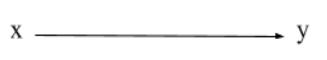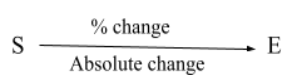# Percentages

## Introduction

The percentage is a very commonly used term, but there is the fact that most of us don't know! The percentage is a word that is derived from the french word 'cent,' which means 100 in french.

Percentage basically is essentially out of hundred. This means that we compare data and numbers revolving around the value “100”.

For example: If we have a class of 6 students (A1, A2, A3, A4, A5, A6) and these six students have appeared for the 12th board exams, then we can judge which student is good in studies by looking at the percentages of them.

And now we can conclude that student A5 is the best out of these as the student has the maximum percentage of marks amongst all the students.

Did you know? Whenever you multiply any ratio with 100, it returns the percentage. But how?

Let’s take the help of the unitary method to know this.

Unitary Method: Whenever we have a situation where two variables move in a linear manner with respect to each other, then the unitary method comes into play.

Why not have an example to understand this method?

Example

Raju goes to the market and buys ten apples for rupees 30. How much will he pay for 15 apples?
Solution

Let’s see how we can implement the unitary method in the percentage-based questions:

Example

8% of a number is 6000. What is the number?

Solution

Now, as we have understood percentage so let's see the concept of the percentage change.

## Percentage Change

The term percentage always occurs when we go from one number to another. But the basic structure of percentage change will only and only occur when we talk about the difference between two numbers

Let’s understand this with an example:

If we have a number x that is changing to ythen the percentage change of x to y will be

Let's see this actual using numbers.

If we have two numbers, 40 and 60, and we are going from 40 to 60, then we will have the percentage change as follows:

But if we have the same two numbers and the change this time is from 60 to 40 then the change is as follows:

NOTE: 1. In percentage change, there should always be two numbers.

2. we need to figure out which number is the original number.

## Percentage Change Graphics

An important topic that is used in chapters like interest, profit, and loss, etc. So basically, when we use a graphical method for doing the percentage change, it is generally called percentage change graphics.

Basics of percentage change

This was simple, wasn’t it?

Now PCG generally has two structures,

Structure 1

Given the starting value and the ending value. We will have to calculate the following two things:

1. Absolute Change
2. % ChangeLet's understand this taking the help of an example :

Example

Structure 2

1. The initial value is given to us.
2. Percentage change is given to us.
3. Absolute change is what we need to calculate.
4. And calculating the ending value as well.

Example

## Problems on Percentage Change

Let’s see some good problems on Percentage change based on different topics.

### Area and volume-based problem

Problem Statement

The length of a rectangle gets increased by 30%, and the breadth is decreased by 10%. What is the percentage change in area?

Solution

### Expenditure and revenue problem

Problem Statement

The price of petrol goes up by 20%, and people reduce its consumption by 10%. What will be the % change in expenditure?

Solution

## Product Constancy

Whenever we end up with the changes in a series, we need to come back to the original value, and this is called product constancy. It is applied in a lot of aptitude questions directly.

Problem Statement

The price of petrol has gone up by 25%, and the consumption of Petrol is reduced such that the expenditure remains constant.

Solution

Since we have understood the percentage, lets take a look at some questions related to the topic, and let's see their solutions as well!

## Some Important Questions

Problem 1: A shopkeeper selling tables reduces the price of tables by 20%, due to which he gets an increment of 60% in the sale. What is the percentage change in the revenue?

Solution

Problem 2: Bob(B) gets 20% more marks than Aman(A), and Chaitanya(C) gets 50% more marks than Bob. Then how much % less than Chaitanya does Aaman get?

Solution

Some frequently asked questions are present too! So let’s have a look at them as well and clear the frequently asked questions now.

Question 1: How do we find the percentage?
On dividing the given value by the total value and then multiplying it by a hundred returns us the percentage as a result.

Question 2: what is percentage change?
The term percentage always occurs when we go from one number to another. But the basic structure of percentage change will only and only occur when we talk about the difference between two numbers.

Question 3: What is product constancy?
Whenever we end up with the changes in a series, we need to come back to the original value, and this is called product constancy. It is applied in a lot of aptitude questions directly.

## Key Takeaways

This blog takes you around how to find Percentages in detail. With this done, you may now switch towards aptitude preparation from our Guided Path.

As we all know, aptitude actually is a very easy way to score in interviews, so topics like this can help you clear aptitude rounds of a lot of interviews.

If you wish to read more blogs on aptitude, you can find spectacular blogs here.

Happy learning!